# The Azimuth Project Blog - network theory (part 24) (Rev #5, changes)

Showing changes from revision #4 to #5: Added | Removed | Changed

This page is a blog article in progress, written by John Baez. To see discussions of this article while it is being written, go to the Azimuth Forum.

Now we’re ready to prove the deficiency zero theorem. First let’s talk about it informally a bit. Then we’ll review the notation, and then give the proof.

The crucial trick is to relate a bunch of chemical reactions, described by a ‘reaction network’ like this“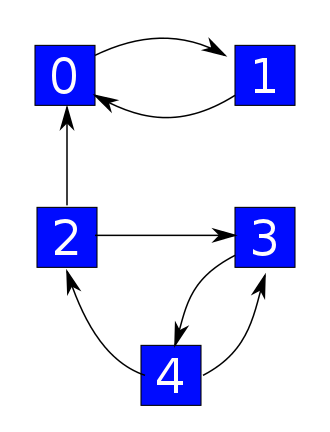to a simpler problem where a system randomly hops between states arranged in the same pattern: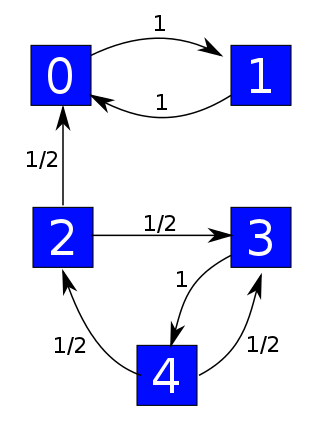This is sort of amazing, because we’ve thrown out lots of detail. It’s also amazing because this simpler problem is linear In the original problem, the chance that a reaction turns an A + C into a B is proportional to the number of A’s times the number of C’s. That’s nonlinear! But in the simplified problem, the chance that your system hops from state 2 to state 0 is just proportional to the probability that it’s in state 2 to begin with. That’s linear.

The wonderful thing is that, at least under some conditions, we can find equilibrium solutions of our original problem starting from equilibrium solutions of the simpler problem.

Let’s roughly sketch how it works, including what we’ve done so far. Our simplified problem is described by an equation like this:

 \displaystyle{ \frac{d}{d t} \psi = H \psi

where $\psi$ describes the probability of being in each state, and $H$ describes the probability of hopping from one state to another. We can fairly easily understand quite a lot about the equilibrium solutions, where

$H \psi = 0$

because this is a linear equation. We did this in Part 23. Of course, when I say ‘fairly easily’, that’s a relative thing: we needed to use the Perron–Frobenius theorem, which Jacob introduced in Part 20. But that’s a well-known theorem in linear algebra, and it’s easy to apply.

In Part 22 we saw that the original problem is described by an equation like this, called the ‘rate equation’:

$\displaystyle{ \frac{d x}{d t} = Y H x^Y }$

Here $x$ is a vector whose entries describe the amount of each kind of chemical: the amount of A’s, the amount of B’s, and so on. The matrix $H$ is the same as in the simplified problem, but $Y$ is a matrix that says how many times each chemical shows up in each spot in our reaction network:The key trick is the funny operation $x^Y$, where we take a vector and raise it to the power of a matrix. This operation makes the equation nonlinear.

We’re looking for equilibrium solutions of the master equation, where the rate of change is zero:

$Y H x^Y = 0$

But in fact we’ll find solutions of

$H x^Y = 0$

since these will automatically obey $Y H x^Y = 0$. And we’ll get these solutions by taking the solutions of

$H \psi = 0$

and showing they

### Review

The storm is brewing for the final climax, so let’s remember where we are. We start with a stochastic reaction network: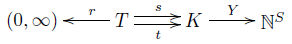This consists of:

• finite sets of transitions $T$, complexes $K$ and species $S$,

• a map $r: T \to (0,\infty)$ giving a rate constant for each transition,

source and target maps $s,t : T \to K$ saying where each transition starts and ends,

• a map $Y : K \to \mathbb{N}^S$ saying how each complex is made of species.

Then we extend $s, t$ and $Y$ to linear maps: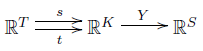Then we put inner products on these vector spaces as described last time, which lets us ‘turn around’ the maps $s$ and $t$ by taking their adjoints:

$s^\dagger, t^\dagger : \mathbb{R}^K \to \mathbb{R}^T$

More surprisingly, we can ‘turn around’ $Y$ and get a nonlinear map using ‘matrix exponentiation’:

$\begin{array}{ccc} \mathbb{R}^S &\to& \mathbb{R}^K \\ x &\mapsto& x^Y \end{array}$

This is most easily understood by thinking of $x$ as a row vector and $Y$ as a matrix:

$\begin{array}{ccl} x^Y &=& {\left( \begin{array}{cccc} x_1 , & x_2 , & \dots, & x_k \end{array} \right)}^{ \left( \begin{array}{cccc} Y_{11} & Y_{12} & \cdots & Y_{1 \ell} \\ Y_{21} & Y_{22} & \cdots & Y_{2 \ell} \\ \vdots & \vdots & \ddots & \vdots \\ Y_{k1} & Y_{k2} & \cdots & Y_{k \ell} \end{array} \right)} \\ \\ &=& \left( x_1^{Y_{11}} \cdots x_k^{Y_{k1}} ,\; \dots, \; x_1^{Y_{1 \ell}} \cdots x_k^{Y_{k \ell}} \right) \end{array}$

Remember, complexes are made out of species. The matrix entry $Y_{i j}$ says how many things of the $j$th species there are in a complex of the $i$th kind. If $\psi \in \mathbb{R}^K$ says how many complexes there are of each kind, $Y \psi \in \mathbb{R}^S$ says how many things there are of each species. Conversely, if $x \in mathbb{R}^S$ says how many things there are of each species, $Y^x \in \mathbb{R}^K$ says how many ways we can build each kind of complex from them.

So, we get these maps: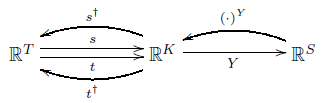Next, the boundary operator

$\partial : \mathbb{R}^T \to \mathbb{R}^K$

describes how each transition causes a change in complexes:

$\partial = t - s$

As we saw last time, there is a Hamiltonian

$H : \mathbb{R}^K \to \mathbb{R}^K$

describing a Markov processes on the set of complexes, given by

$H = \partial s^\dagger$

But the star of the show is the rate equation. This describes how the number of things of each species changes with time. We write these numbers in a list and get a vector $x \in \mathbb{R}^S$ with nonnegative components. The rate equation says:

$\displaystyle{ \frac{d x}{d t} = Y H x^Y }$

We can read this as follows:

$x$ says how many things of each species we have now.

$x^Y$ says how many complexes of each kind we can build from these species.

$s^\dagger x^Y$ says how many transitions of each kind can originate starting from these complexes, with each transition weighted by its rate.

$H x^Y = \partial s^\dagger x^Y$ is the rate of change of the number of complexes of each kind, due to these transitions.

$Y H x^Y$ is the rate of change of the number of things of each species.

### The zero deficiency theorem

We are looking for equilibrium solutions of the rate equation, where the number of things of each species doesn’t change at all:

$Y H x^Y = 0$

In fact we will find complex balanced equilibrium solutions, where even the number of complexes of each kind don’t change:

$H x^Y = 0$

More precisely, we have:

Deficiency Zero Theorem (Child’s Version). Suppose we have a reaction network obeying these two conditions:

1. It is weakly reversible, meaning that whenever there’s a transition from one complex $\kappa$ to another $\kappa'$, there’s a directed path of transitions going back from $\kappa'$ to $\kappa$.

2. It has deficiency zero, meaning $\mathrm{im} \partial \cap \mathrm{ker} Y = \{ 0 \}$.

Then for any choice of rate constants there exists a complex balanced equilibrium solution of the rate equation where all species are present in nonzero amounts. In other words, there exists $x \in \mathbb{R}^S$ with all components positive and such that:

$H x^Y = 0$

Proof. Because our reaction network is weakly reversible, the theorems in Part 23 show there exists $\psi \in (0,\infty)^K$ with

$H \psi = 0$

This $\psi$ may not be of the form $x^Y$, but we shall adjust $\psi$ so that it becomes of this form, while still remaining a solution of $H \psi = 0$. To do this, we need a couple of lemmas:

Lemma 1. $\ker \partial^\dagger + \im Y^\dagger = \mathbb{R}^K$.

Proof. We need to use a few facts from linear algebra. If $V$ is a finite-dimensional vector space with inner product, the orthogonal complement $L^\perp$ of a subspace $L \subseteq V$ consists of vectors that are orthogonal to everything in $L$:

$L^\perp = \{ v \in V : \quad \forall w \in L \quad \langle v, w \rangle = 0 \}$

We have

$(L \cap M)^\perp = L^\perp + M^\perp$

where $L$ and $M$ are subspaces of $V$ and $+$ denotes the sum of subspaces. Also, if $T: V \to W$ is a linear map between finite-dimensional vector spaces with inner product, we have

$(\mathrm{ker} T)^\perp = \mathrm{im} T^\dagger$

and

$(\mathrm{im} T)^\perp = \mathrm{ker} T^\dagger$

Now, because our reaction network has deficiency zero, we know that

$\mathrm{im} \partial \cap \mathrm{ker} Y = \{ 0 \}$

Taking the orthogonal complement of this subspace of $\mathbb{R}^K$, we get

$(\mathrm{im} \partial \cap \mathrm{ker} Y)^\perp = \mathbb{R}^K$

and using the rules we mentioned, we obtain

$\ker \partial^\dagger + \im Y^\dagger = \mathbb{R}^K$

as desired.   █

Now, given a vector $\phi$ in $\mathbb{R}^K$ or $\mathbb{R}^S$ with all positive components, we can define the logarithm of such a vector, componentwise:

$(\ln \phi)_i = \ln (\phi_i)$

Similarly, for any vector $\phi$ in either of these spaces, we can define its exponential in a componentwise way:

$(\exp \phi)_i = \exp(\phi_i)$

These operations are inverse to each other. Moreover:

Lemma 2. The nonlinear operator

$\begin{array}{ccc} \mathbb{R}^S &\to& \mathbb{R}^K \\ x &\mapsto& x^Y \end{array}$

is related to the linear operator

$\begin{array}{ccc} \mathbb{R}^S &\to& \mathbb{R}^K \\ x &\mapsto& Y^\dagger x \end{array}$

by the formula

$x^Y = \exp(Y^\dagger \ln x )$

which holds for all $x \in (0,\infty)^S$.

Proof. A straightforward calculation. By the way, this formula would look a bit nicer if we treated $\ln x$ as a row vector and multiplied it on the right by $Y$: then we would have

$x^Y = \exp((\ln x) Y)$

The problem is that we are following the usual convention of multiplying vectors by matrices on the left, yet writing the matrix on the right in $x^Y$; in a sense the transpose $Y^\dagger$ of the matrix $Y$ serves to compensate for this.   █

Now, given our vector $\psi \in (0,\infty)^K$ with $H \psi = 0$, we obtain $\ln \psi \in \mathbb{R}^K$. Lemma 1 says that

$\mathbb{R}^K \in \ker \partial^\dagger + \im Y^\dagger$

so we can write

$\ln \psi = \alpha + Y^\dagger \beta$

for some $\alpha \in \ker \partial^\dagger$ and some $\beta \in \mathbb{R}^S$. Moreover, we can write

$\beta = \ln x$

for some $x \in (0,\infty)^S$, so that

$\ln \psi = \alpha + Y^\dagger (\ln x)$

Exponentiating both sides componentwise, we get

$\psi = \exp(\alpha) \; \exp(Y^\dagger (\ln x))$

where at right we are taking the componentwise product of vectors. Thanks to Lemma 2, we conclude that

$\psi = \exp(\alpha) x^Y$

So, we have taken $\psi$ and almost written it in the form $x^Y$—but not quite! We can adjust $\psi$ to make it be of this form:

$\exp(-\alpha) \psi = x^Y$

Clearly all the components of $\exp(-\alpha) \psi$ are positive, since the same is true for both $\psi$ and $\exp(-\alpha)$. So, the only remaining task is to check that $\exp(-\alpha) \psi$ is still a complex balanced equilibrium solution of the rate equation:

Lemma 3. If $H \psi = 0$ and $\alpha \in \ker \partial^\dagger$, then $H(\exp(-\alpha) \psi) = 0$.

Proof. It is enough to check that multiplication by $\exp(-\alpha)$ commutes with the Hamiltonian $H$, since then

$H(\exp(-\alpha) \psi) = \exp(-\alpha) H \psi = 0$

Recall from Part 23 that $H$ is the Hamiltonian of a Markov process associated to this ‘graph with rates’: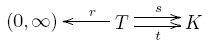As noted here:

• John Baez and Brendan Fong, A Noether theorem for Markov processes.

multiplication by a function on $K$ commutes with $H$ if and only if that function is constant on each connected component of this graph. So, it suffices to show that $\exp(-\alpha)$ is constant on each connected component. For this, it is enough to show that $\alpha$ itself is constant on each connected component. But this follows from the next lemma, since $\alpha \in \ker \partial^\dagger$.   █

Lemma 4. A function $\alpha \in \mathbb{R}^K$ is constant on each connected component of the graph $s, t: T \to K$ iff $\partial^\dagger \alpha = 0$

Proof. Suppose $\partial^\dagger \alpha = 0$, or in other words, $\alpha \in \mathrm{ker} \partial^\dagger$, or in still other words, $\alpha \in (\mathrm{im} \partial)^\perp$. To show that $\alpha$ is constant on each connected component, it suffices to show that whenever we have two complexes connected by a transition, like this:

$\tau: \kappa \to \kappa'$

then $\alpha$ takes the same value at both these complexes:

$\alpha_\kappa = \alpha_{\kappa'}$

To see this, note

$\partial \tau = t(\tau) - s(\tau) = \kappa' - \kappa$

and since $\alpha \in (\mathrm{im} \partial)^\perp$, we conclude

$\langle \alpha, \kappa' - \kappa \rangle = 0$

But calculating this inner product, we see

$\alpha_{\kappa'} - \alpha_{\kappa} = 0$

as desired.

For the converse, we simply turn the argument around: if $\alpha$ is constant on each connected component, we see $\langle \alpha, \kappa' - \kappa \rangle = 0$ whenever there is a transition $\tau : \kappa \to \kappa'$. It follows that $\langle \alpha, \partial \tau \rangle = 0$ for every transition $\tau$, so $\alpha \in (\im \partial)^\perp$.

And thus concludes the proof of the lemma!   █

And thus concludes the proof of the theorem!   █

And thus concludes this post!

category: blog